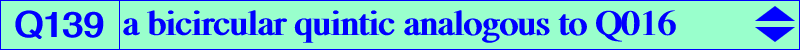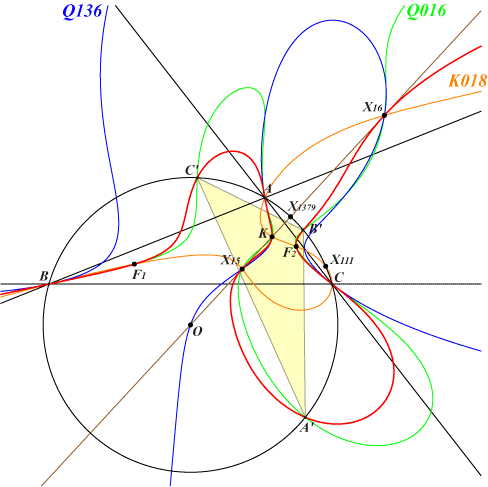too complicated to be written here. Click on the link to download a text file.X(6), X(15), X(16), X(524) A'B'C' : circumcevian triangle of X(6) foci of the Steiner inellipse anti-points, see Table 77Q139 is mentioned by Angel Montesdeoca in Hyacinthos #26311. Q139 is a bicircular quintic very similar to the Lemoine quintic Q016 and their 25 common points are A, B, C (counting for 6 since the common tangents are the symmedians), A', B', C', X(6), X(15), X(16), X(524) (counting for 3), the 4 foci of the Steiner inellipse, the circular points at infinity (each counting for 3). These two quintics generate a pencil which contains the union of K018 and the circumcircle. A, B, C are points of inflexion on Q139. The Brocard axis is bitangent at X(15), X(16) to Q139. The tangents at the real foci of the Steiner inellipse pass through X(1379) hence they are the same as those of the Thomson cubic K002 at these points. The 4th polar curve of X(6) is Q136. It is the locus of points whose polar line in Q139 passes through X(6). K018 is the locus of points whose polar conic in Q139 is a rectangular hyperbola. In other words, K018 is the Laplacian of Q139.